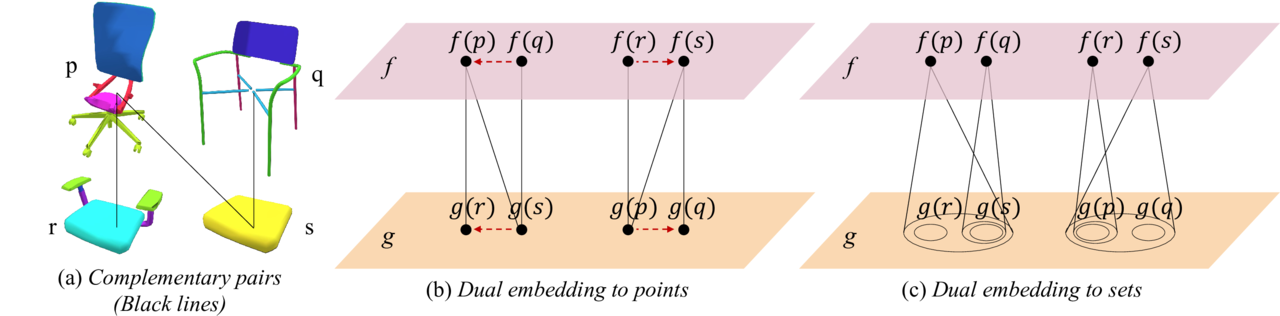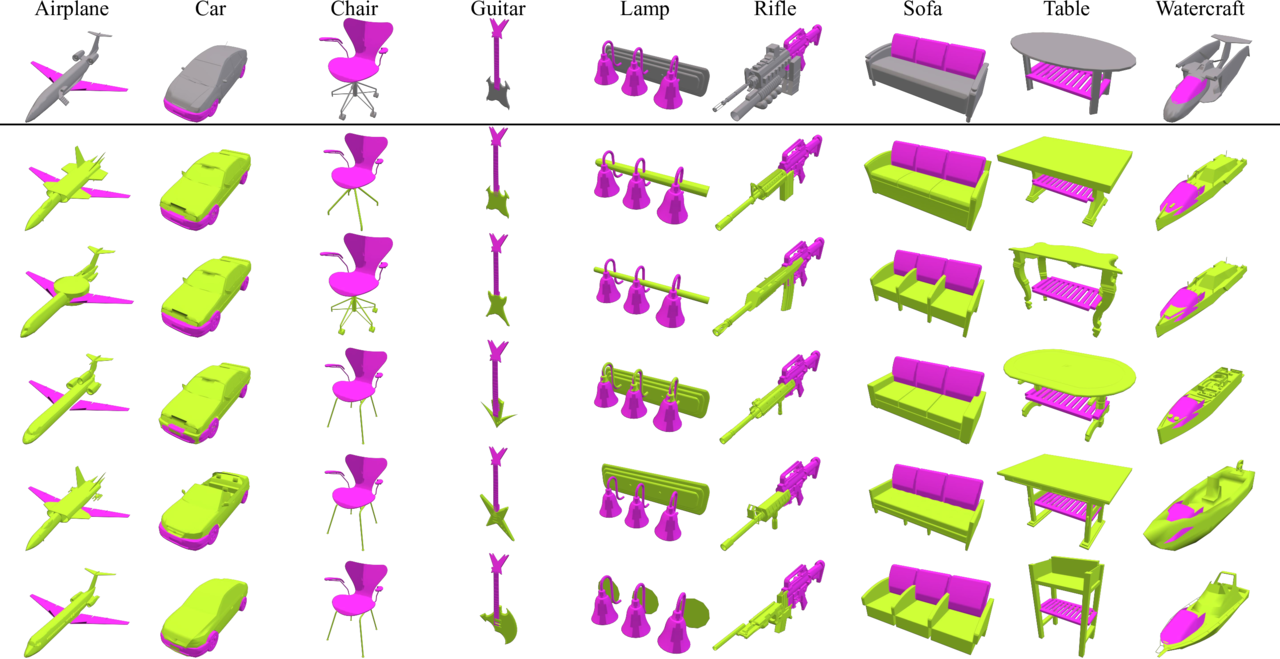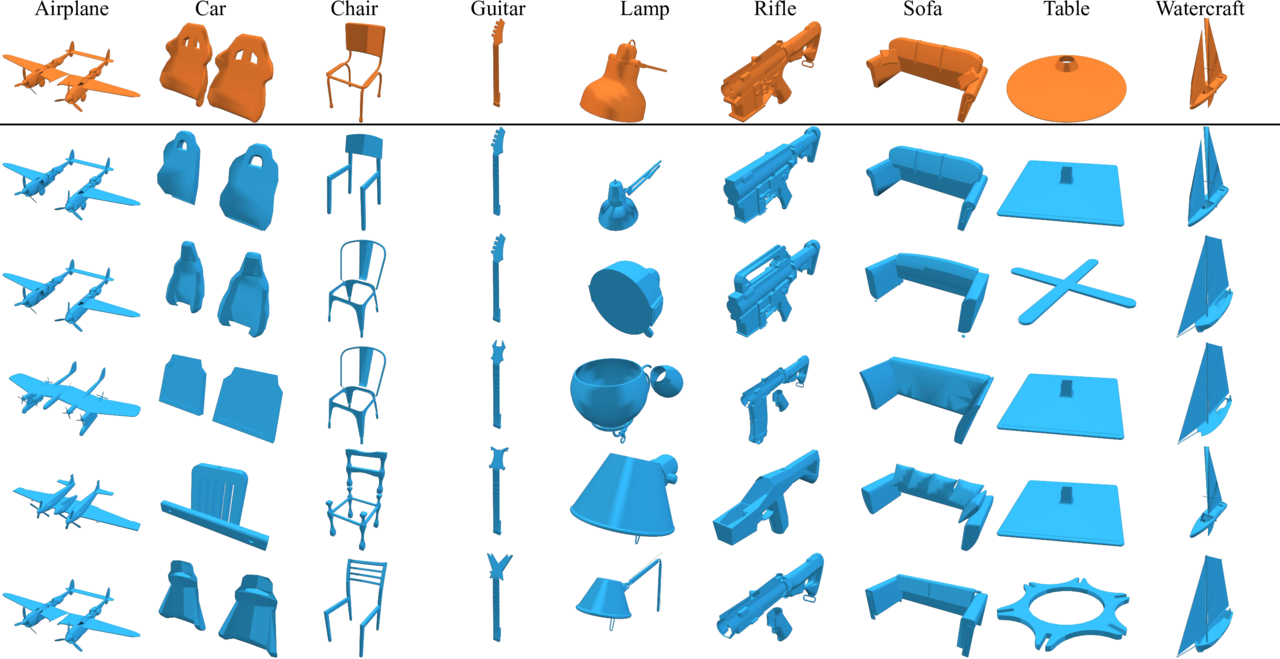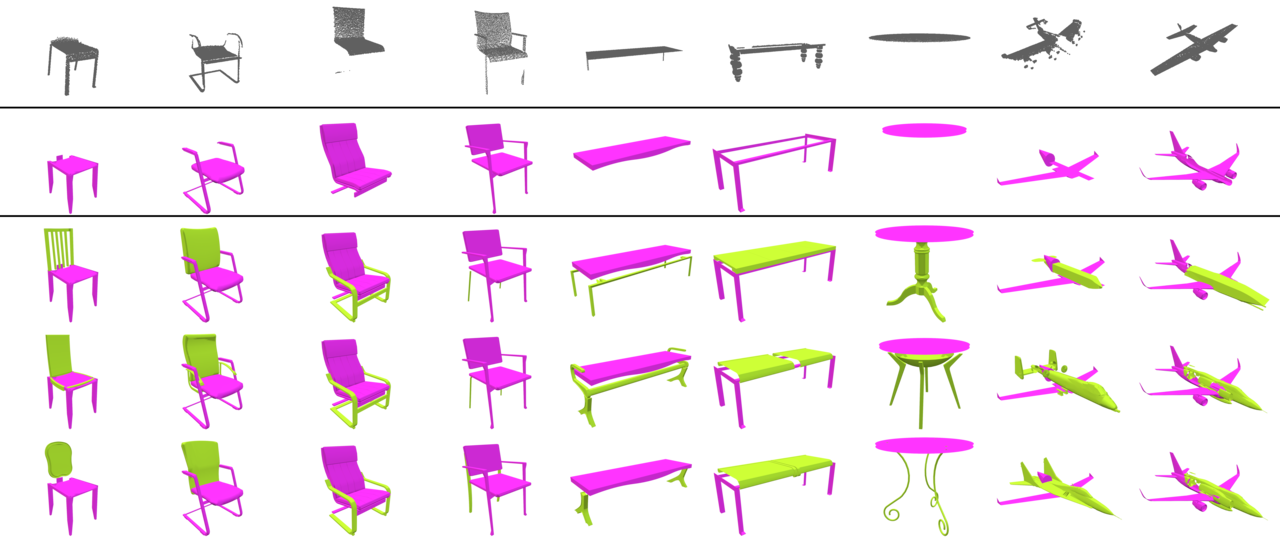## Learning Fuzzy Set Representations of Partial Shapes on Dual Embedding Spaces

#### SGP 2018 (Symposium on Geometry Processing)### Abstract

Modeling relations between components of 3D objects is essential for many geometry editing tasks. Existing techniques commonly rely on labeled components, which requires substantial annotation effort and limits components to a dictionary of pre-defined semantic parts. We propose a novel framework based on neural networks that analyzes an uncurated collection of 3D models from the same category and learns two important types of semantic relations among full and partial shapes: complementarity and interchangeability. The former helps to identify which two partial shapes make a complete plausible object, and the latter indicates that interchanging two partial shapes from different objects preserves the object plausibility. Our key idea is to jointly encode both relations by embedding partial shapes as fuzzy sets in dual embedding spaces. We model these two relations as fuzzy set operations performed across the dual embedding spaces, and within each space, respectively. We demonstrate the utility of our method for various retrieval tasks that are commonly needed in geometric modeling interfaces.

Minhyuk Sung, Anastasia Dubrovina, Vladimir G. Kim, and Leonidas Guibas
Learning Fuzzy Set Representations of Partial Shapes on Dual Embedding Spaces
SGP 2018 (Symposium on Geometry Processing)

arXiv | Paper | Slides(PPTX) | Code

### Bibtex

@article{Sung:2018,
author = {Sung, Minhyuk and Dubrovina, Anastasia and Kim, Vladimir G. and Guibas, Leonidas},
title = {Learning Fuzzy Set Representations of Partial Shapes on Dual Embedding Spaces},
Journal = {Computer Graphics Forum (Proc. of Symposium on Geometry Processing (SGP))},
year = {2018}
}


### Overview & ResultsFig. 3. A schematic example showing how our set representation works on the dual embedding space. (a) $$(p, r)$$ $$(p, s)$$, and $$(q, s)$$ are complementary pairs, and $$(q, r)$$ is a non-complementary pair. (b) A case of embedding data as points, and aligning embedding coordinates of complementary shapes in different spaces (e.g. $$f(p) = g(s)$$ and $$f(r) = g(p)$$). The complementarity of $$(p,r)$$ and $$(p,s)$$ implies that $$g(r) = g(s)$$ (similarly, $$f(r) = f(s)$$). Because of the complementarity of $$(q, s)$$ this also incorrectly implies that $$g(q) = g(r)$$ ($$f(q) = f(r)$$), which results in a wrong complementary relation between $$q$$ and $$r$$. (c) The proposed approach of representing complementarity with set representations. All relations can be correctly encoded.Fig. 5. Examples of top-5 complement shape retrievals. The top row shows the original shapes (gray) with highlighted query partial shapes (magenta). Next five rows show the retrieved top-5 complementary partial shapes (green), together with the query shapes (magenta). See the accompanying text for details.Fig. 6. Examples of partial shape interchangeability. The top row shows the query partial shapes. Next five rows show partial shapes interchangeable with it, which were detected using the proposed approach. Different components comprising the partial shapes are shown with different shades of blue. See the accompanying text for details.Fig. 10. Examples of partial scan completion. We used synthetic partial scan data from the benchmark of [Sung et al. 2015] (first row). We first use ICP to retrieve the best fitting partial shape from our database (second row, colored in pink), and then complete it to a full shape using various complements retrieved by the proposed method (colored in green). Three out of the best ten completions are shown in third, fourth, and fifth rows. The positions of the complements are automatically predicted using the placement network of [Sung et al. 2017].

#### Acknowledgements

This project was supported by NSF grants IIS-1528025 and DMS-1521608, MURI award N00014-13-1-0341, a Google focused research award, the Korea Foundation for Advanced Studies, and gifts from the Adobe systems and Autodesk corporations.